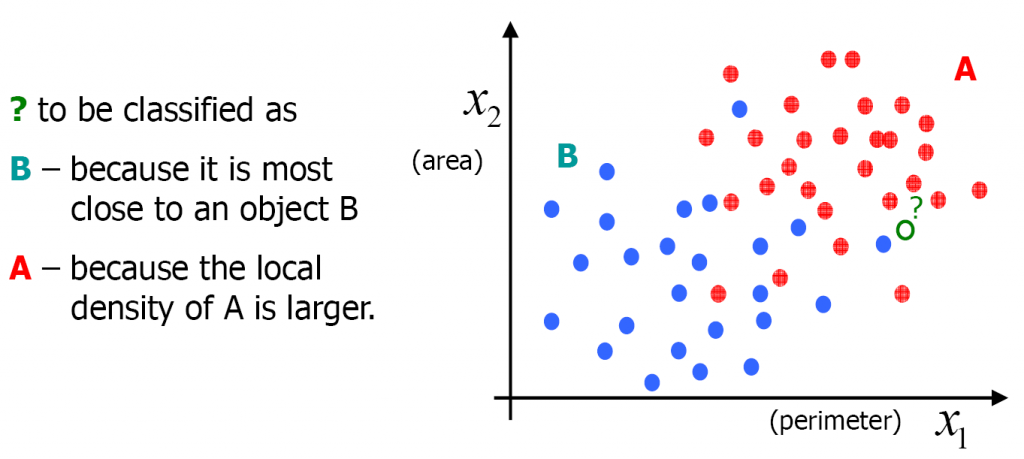What is the ground for classifying a new object if we just have some some examples?
How to we determine a proper class given a training set?

We may find an identical copy in the examples if we are lucky. If there are more identical copies and they belong to different classes we are in trouble. Now it depends on how the set of examples has been constructed. If the set is representative for the problem in the probabilistic sense a majority vote will be the way to proceed.

But what to do in case all objects are different as for most real world objects?

### All objects are different, how to generalize?

If the examples are all different from the new object and we want to use them for classifying it, they should be related. It is then necessary to find an object representation that enables us to relate them. By this a distance or a similarity may be obtained for the new object to all objects in the training set. What to do now? What will be the next step after for instance all distances have been obtained. There are two directions to obtain an answer.### Distances

First we may have a look at the most similar object in the examples, the nearest neighbor. Its class may be used for classifying the new object. All other objects in the set  are neglected, just the nearest one is used. An argument for that is that real world objects that are similar are expected to belong to the same class to have a similar representation. Objects that are very different will belong to different classes and have very different representations. So classifying by the nearest neighbor distance is a natural way to do. This assumes that the representation satisfies some continuous properties: small object differences will cause small changes in the representation.

### Densities

A different way to find an answer is that we not just consider just the nearest neighbor but a set of neighbors, eventually all objects. Their distances may be weighted and result in a probability density estimate for each of the classes for the object under consideration. This is called a density kernel approach, or a Parzen density estimation. Instead some density model may be used, e.g. a normal distribution. In both ways the training set is used to build statistical models of the classes. New objects are assigned to the most probable class according to these models, possibly taken class priors into account.

### Both

Distances may be considered as a local approach and densities as global. In practice most classification rules make use of both principles, with a few exceptions. Distance based classifiers like the Support Vector Machine that take class overlap into account have somewhere a probabilistic trade-off. Density based classifiers on the other hand, need to estimate densities. Density estimators are depend on the metric and thereby on distances.

In studying the characteristics of classifiers it can be very helpful to trace where and in what way the classifier makes use of distances or densities. There are a few exceptions. One is the decision tree which is based on just a ranking of distances.

### In conclusion: distances are crucial and densities may help in addition

Filed under: ClassificationFoundationPR System